• 椭圆曲线加密算法 椭圆曲线加密算法，即：Elliptic Curve Cryptography，简称ECC，是基于椭圆曲线数学理论实现的一种非对称加密算法。相比RSA，ECC优势是可以使用更短的密钥，来实现与RSA相当或更高的安全。据研究，...
椭圆曲线加密算法
椭圆曲线加密算法，即：Elliptic Curve Cryptography，简称ECC，是基于椭圆曲线数学理论实现的一种非对称加密算法。相比RSA，ECC优势是可以使用更短的密钥，来实现与RSA相当或更高的安全。据研究，160位ECC加密安全性相当于1024位RSA加密，210位ECC加密安全性相当于2048位RSA加密。
一般椭圆曲线方程式表示为:(其中a,b,c,d为系数)
> y2=ax3+ bx2+cx+d
典型的椭圆曲线如：y2=x3−4x2+16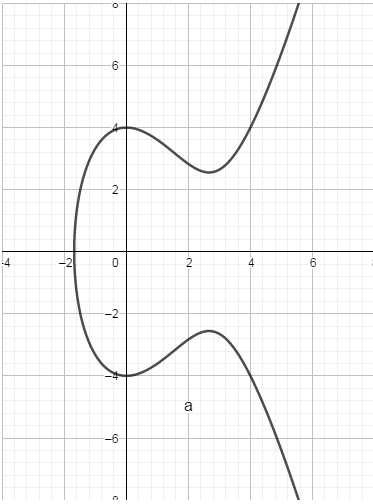先摆一个栗子：
小米只会加法，不会乘法，一天小王问小米。

小王: 你知道几个5相加等于15？
小米: 3个。
小王：再考考你，几个5相加等于55？
小米：算了半天，终于算出来了，11对吧。
小王: 我就不行考不到你，那‭390625呢？
小米：.............‬

小米很难算到的那个数，就是公钥密码算法中的私钥（一个公钥密码算法安全的必要条件（非充分）是“由公钥不能反推出私钥”），公钥密码算法最根本的原理是利用信息的不对称性：即掌握私钥的人在整个通信过程中掌握最多的信息。
椭圆曲线加密算法是一个基于加法阶数难求问题的密码方案。 对于椭圆曲线来讲，椭圆曲线的基点就是例子里面的5，而私钥就是基点的加法阶数（例子里面的11），公钥是基点（5）进行对应阶数的加法（11次）得到的结果（55）。
简单描述就是:G * k = K （G,K公开，k保密）

k就是传说中的密钥
K就是传说中的公钥
G一般在算法里写死了，也叫做曲线参数(例子中的G点)

上述例子相对简单，椭圆曲线加密算法里的加法建立在 “有限域上的二元三次曲线上的点”上 ，组成一个“有限加法循环群”。具体的说，这个加法的几何定义如下图，两个点的加法结果是指这两点的连线和曲线的交点关于x轴的镜像。

加法 ：过曲线上的两点A、B画一条直线，找到直线与椭圆曲线的交点，交点关于x轴对称位置的点，定义为A+B，即为加法。如下图所示：A + B = C。

如果A=B时，即两点重合的情况，将椭圆曲线在A点的切线，与椭圆曲线的交点，交点关于x轴对称位置的点，定义为A + A，即2A。如图: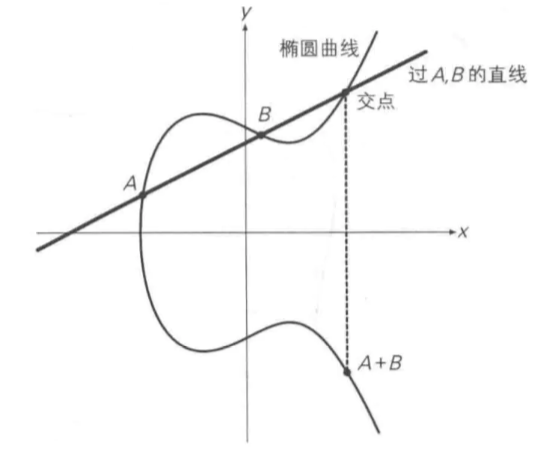将A关于x轴对称位置的点定义为-A，即椭圆曲线的正负取反运算,如图: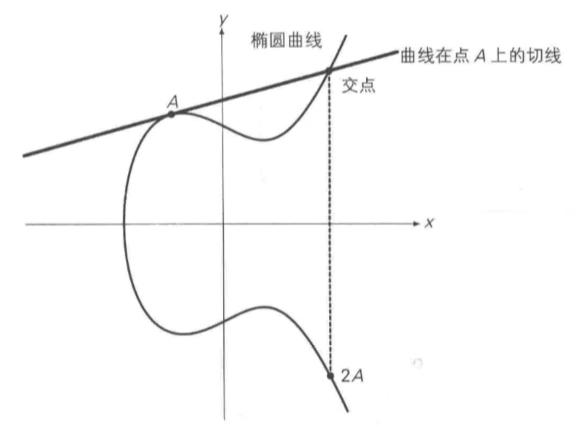如果我们从某一点出发（所谓的单位元，比如正整数域的1，代表一个空间里的最基本单元），不停做自增操作（所谓群操作，比如++），枚举出整个空间的集合元素。如图：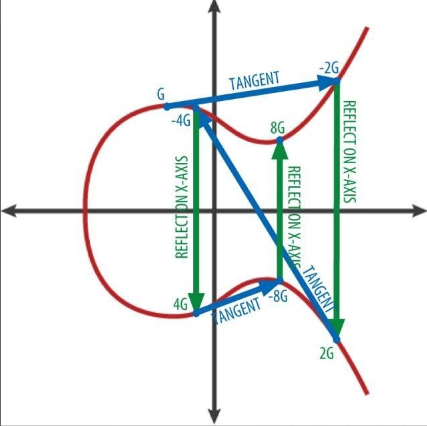因此给定椭圆曲线的某一点G，从G出发，不停做切线，找对称点，依次得到-2G，2G，-4G，4G，-8G，8G… 。即：当给定G点时，已知x，求xG点并不困难。反之，已知xG点，求x则非常困难。即Q = NG，N就是我们的私钥，Q就是我们的公钥。


展开全文• 椭圆曲线加密算法的 C 语言设计和实现 椭圆曲线加密算法于 1985 年提出由于自身优点它一出现便受到关注现在密码学界普 遍认为它将替代 RSA 加密算法 成为通用的公钥加密算法那么我们今天就来看看椭圆曲线 加密算法是...
• 基于国家商用密码开放动态库开发的椭圆曲线加密算法密钥生成器
• 椭圆曲线加密算法的发展趋势.椭圆曲线加密算法的发展趋势.pdf
• 椭圆曲线加密算法程序完整版 VC++ VC6的工程。可用
• 为了解决应用于嵌入式系统的椭圆曲线加密算法存在的加密速度较慢、系统开销过大等问题，改善其算法性能，提高加密速度，减少系统开销，对实际研发的嵌入式密码器中应用的椭圆曲线加密算法做了深入分析，并在此基础上...
• 椭圆曲线加密算法用C++完整实现，很好的东西！！
• 椭圆曲线加密算法，即：Elliptic Curve Cryptography，简称ECC，是基于椭圆曲线数学理论实现的一种非对称加密算法。相比RSA，ECC优势是可以使用更短的密钥，来实现与RSA相当或更高的安全。据研究，160位ECC加密安全...
椭圆曲线加密算法，即：Elliptic Curve Cryptography，简称ECC，是基于椭圆曲线数学理论实现的一种非对称加密算法。相比RSA，ECC优势是可以使用更短的密钥，来实现与RSA相当或更高的安全。据研究，160位ECC加密安全性相当于1024位RSA加密，210位ECC加密安全性相当于2048位RSA加密。
椭圆曲线在密码学中的使用，是1985年由Neal Koblitz和Victor Miller分别独立提出的。

椭圆曲线
一般情况下，椭圆曲线可用下列方程式来表示，其中a,b,c,d为系数。

E:y2=ax3+ bx2+cx+d

例如，当a=1,b=0,c=-2,d=4时，所得到的椭圆曲线为:

E:y2=x3-2x+4

该椭圆曲线E的图像如图X-1所示，可以看出根本就不是椭圆形。定义椭圆曲线的运算规则
加法
过曲线上的两点A、B画一条直线，找到直线与椭圆曲线的交点，交点关于x轴对称位置的点，定义为A+B，即为加法。如下图所示：A + B = C二倍运算
上述方法无法解释A + A，即两点重合的情况。因此在这种情况下，将椭圆曲线在A点的切线，与椭圆曲线的交点，交点关于x轴对称位置的点，定义为A + A，即2A，即为二倍运算。正负取反
将A关于x轴对称位置的点定义为-A，即椭圆曲线的正负取反运算。如下图所示：无穷远点
如果将A与-A相加，过A与-A的直线平行于y轴，可以认为直线与椭圆曲线相交于无穷远点。
综上，定义了A+B、2A运算，因此给定椭圆曲线的某一点G，可以求出2G、3G（即G + 2G）、4G……。即：当给定G点时，已知x，求xG点并不困难。反之，已知xG点，求x则非常困难。此即为椭圆曲线加密算法背后的数学原理。
有限域上的椭圆曲线运算
椭圆曲线要形成一条光滑的曲线，要求x,y取值均为实数，即实数域上的椭圆曲线。但椭圆曲线加密算法，并非使用实数域，而是使用有限域。按数论定义，有限域GF(p)指给定某个质数p，由0、1、2……p-1共p个元素组成的整数集合中定义的加减乘除运算。
假设椭圆曲线为y² = x³ + x + 1，其在有限域GF(23)上时，写作：
y² ≡ x³ + x + 1 (mod 23)
此时，椭圆曲线不再是一条光滑曲线，而是一些不连续的点，如下图所示。以点(1,7)为例，7² ≡ 1³ + 1 + 1 ≡ 3 (mod 23)。如此还有如下点：
(0,1) (0,22)
(1,7) (1,16)
(3,10) (3,13)
(4,0)
(5,4) (5,19)
(6,4) (6,19)
(7,11) (7,12)
(9,7) (9,16)
(11,3) (11,20)
等等。
另外，如果P(x,y)为椭圆曲线上的点，则-P即(x,-y)也为椭圆曲线上的点。如点P(0,1)，-P=(0,-1)=(0,22)也为椭圆曲线上的点。计算xG
相关公式如下：
有限域GF(p)上的椭圆曲线y² = x³ + ax + b，若P(Xp, Yp), Q(Xq, Yq)，且P≠-Q，则R(Xr,Yr) = P+Q 由如下规则确定：
Xr = (λ² - Xp - Xq) mod p
Yr = (λ(Xp - Xr) - Yp) mod p
其中λ = (Yq - Yp)/(Xq - Xp) mod p（若P≠Q）, λ = (3Xp² + a)/2Yp mod p（若P=Q）
因此，有限域GF(23)上的椭圆曲线y² ≡ x³ + x + 1 (mod 23)，假设以(0,1)为G点，计算2G、3G、4G…xG等等，方法如下：
计算2G：
λ = (3x0² + 1)/2x1 mod 23 = (1/2) mod 23 = 12
Xr = (12² - 0 - 0) mod 23 = 6
Yr = (12(0 - 6) - 1) mod 23 = 19
即2G为点(6,19)
计算3G：
3G = G + 2G，即(0,1) + (6,19)
λ = (19 - 1)/(6 - 0) mod 23 = 3
Xr = (3² - 0 - 6) mod 23 = 3
Yr = (3(0 - 3) - 1) mod 23 = 13
即3G为点(3, 13)
同理计算4G、5G…xG，分布如下图：

[图片上传失败…(image-2d5c43-1526642990683)]

椭圆曲线加解密算法原理
建立基于椭圆曲线的加密机制，需要找到类似RSA质因子分解或其他求离散对数这样的难题。而椭圆曲线上的已知G和xG求x，是非常困难的，此即为椭圆曲线上的的离散对数问题。此处x即为私钥，xG即为公钥。
椭圆曲线加密算法原理如下：
设私钥、公钥分别为k、K，即K = kG，其中G为G点。
公钥加密：
选择随机数r，将消息M生成密文C，该密文是一个点对，即：
C = {rG, M+rK}，其中K为公钥
私钥解密：
M + rK - k(rG) = M + r(kG) - k(rG) = M
其中k、K分别为私钥、公钥。
椭圆曲线签名算法原理
椭圆曲线签名算法，即ECDSA。
设私钥、公钥分别为k、K，即K = kG，其中G为G点。
私钥签名：
1、选择随机数r，计算点rG(x, y)。
2、根据随机数r、消息M的哈希h、私钥k，计算s = (h + kx)/r。
3、将消息M、和签名{rG, s}发给接收方。
公钥验证签名：
1、接收方收到消息M、以及签名{rG=(x,y), s}。
2、根据消息求哈希h。
3、使用发送方公钥K计算：hG/s + xK/s，并与rG比较，如相等即验签成功。
原理如下：
hG/s + xK/s = hG/s + x(kG)/s = (h+xk)G/s
= r(h+xk)G / (h+kx) = rG
代码实现：
package main
import (
"crypto/ecdsa"
"crypto/elliptic"
"crypto/rand"
"crypto/sha256"
"math/big"
"fmt"
)
//通过椭圆曲线完成签名和验证
func main() {
//声明明文
message := []byte("hello world")
//生成私钥
//生成公钥
pub := privateKey.PublicKey
//将明文散列
digest := sha256.Sum256(message)
//签名
r, s, _ := ecdsa.Sign(rand.Reader, privateKey, digest[:])
//设置私钥的参数类型为曲线类型
param := privateKey.Curve.Params()
//获得私钥byte长度
curveOrderByteSize := param.P.BitLen() / 8
//获得签名返回值的字节
rByte, sByte := r.Bytes(), s.Bytes()
//创建数组
signature := make([]byte, curveOrderByteSize*2)
//通过数组保存了签名结果的返回值
copy(signature[curveOrderByteSize-len(rByte):], rByte)
copy(signature[curveOrderByteSize*2-len(sByte):], sByte)
//认证
//将明文做hash散列，为了验证的内容对比
digest = sha256.Sum256(message)
curveOrderByteSize = pub.Curve.Params().P.BitLen() / 8
//创建两个整形对象
r, s = new(big.Int), new(big.Int)
//设置证书值
r.SetBytes(signature[:curveOrderByteSize])
s.SetBytes(signature[curveOrderByteSize:])

​
//认证
e := ecdsa.Verify(&pub, digest[:], r, s)

if e == true {
fmt.Println("OK")
} else {
fmt.Println("failed")
}
​

}


展开全文• 椭圆曲线加密算法于1985年提出，由于自身优点，它一出现便受到关注，现在密码学界普遍认为它将替代RSA加密算法成为通用的公钥加密算法。那么我们今天就来看看椭圆曲线加密算法是如何通过C语言来设计实现的。一、椭圆...
椭圆曲线加密算法于1985年提出，由于自身优点，它一出现便受到关注，现在密码学界普遍认为它将替代RSA加密算法成为通用的公钥加密算法。那么我们今天就来看看椭圆曲线加密算法是如何通过C语言来设计实现的。一、椭圆曲线加密算法的C语言设计1、椭圆曲线加密系统的基本结构椭圆曲线的加解密流程如图1所示：椭圆曲线进行加密通信的过程如下：首先选定一个适合加密的椭圆曲线Ep(a，b)，并取椭圆曲线上的一点作为基点G。选择一个私有密钥k，并生成公开密钥K=kG。加密时，将明文编码到Ep(a，b)上的一点M，并产生一个随机整数r(r<n)。计算点C1=M+rK，C2=rGo将C1、C2存入密文。解密时，从密文中读出CI、C2，计算C1-kC2，根据C1-kC2=M+rK-k( rG)=M+rK-r( kG)=M，解得的结果就是点M，即明文。2、高精度整数的表示加密算法几乎都是建立在高精度大整数的运算上， 而一般的程序语言都不提供大整数的结构，因此要表示上百位的高精度整数需另辟蹊径。本文使用了LibTomMath库的高精度整数结构。LibTomMath是一个计算高精度整数的库的开源软件，由加拿大人汤姆St.丹尼斯编写，用标准C语言写了几乎所有标准的密码算法模块，并且在几乎所有的操作系统下都可执行。LibTomMath库对高精度大整数的表示是该库最大的一个特点。在LibTomMath库中的高精度大整数表示如下：在32位机上unsigned long为32bit，用mp_digit表示这个类型：typedef unsigned_long mp_digit；实际使用了32位的28位，少用4位，因此用16进制表示一个mp_digit为0XXXXXXX，其中X为16进制数字，将这个32位bit串称为一个mp_digit单元，若干个mp_digit单元构成一个大整数，结构定义一个大整数mpint如下：typeclef struct {inl used，alloc，sign；mp_digit *dp;) mp_int；其中：dp是存放大整数的地址，将大整数（二进比特串）分段（mp_digit单元）存放在从该地址起的内存里，缺省时分配dp为MP_PREC=64个mp_digit单元，即alloc =64；used为实际使用的mp_digit单元；sign=0表示非负数，为1表示负数。对于分配了alloc个mp_digit的大整数mpjnL，因为实际可以使用的比特数是28*alloc，因此可以表示的整数范围是[-228*alloc，228*alloc]。对于64位机情况类似。3、椭圆曲线的参数选取在基于椭圆曲线的加密和解密实现方案中，首先要给出椭圆曲线域的参数来确定一条椭圆曲线。在SECI及IEEE P1363ECC工作草案中，所定义的二进制域上椭圆曲线用到六个参量T=(p，a，b，G，n，h)o p，a，b用来确定一条椭圆曲线，G为基点，n为点G的阶，h是椭圆曲线上所有点的个数m与n相除的整数部分，这几个参量取值的选择直接影响加密的安全性。参量值一般要求满足以下几个条件：a）p当然越大越安全，但越大，计算速度会变慢，200位左右可以满足一般安全要求；b）p≠nxh；c）pt≠l (mod n)，l≤t<20；d）a3+27b2≠O (mod p)；e）n为素数；f）h≤4。本文选用大素数域上的椭圆曲线E(p)：y2=x3+ax+b作为我们的加密曲线。（1）参数a、b的选取采用构造法产生椭圆曲线CM(Complex Multiplication)法，即先确定有限域Fp和其上的椭圆fHj线的阶，然后再构造满足要求的椭圆曲线，即求出椭圆曲线方程E(p)：y2=x3+ax+b中的a，b。（2）基点的确定首先在椭圆曲线上随机选择一个有效点，然后根据选择的点得到阶是n的有效基点。这里有效基点的阶最好是曲线阶的点，至少是曲线阶的最大素因子，这样可以保证一定的安全。（3）私钥的确定随机选取1到P-1之间的素数作为私钥d。（4）公钥的确定由d乘我们所确定的基点得到公钥K，即K=dG。4、椭圆曲线的点加和标量乘对于一般的椭圆曲线方程yz+alxy+a3y =X3+a2xz+a,x+a6，设点P(xi，yl)，Q(X2，yz)的和R(X3，y3)的坐标为x3=k2+kal+a2+xl+x2；y3=k(XI-X4) -y1-alx4-a3，其中当P≠Q时（点加运算）k=（y1-y2）／（x1-x2）；当P=Q时（倍点运算）k= (3×2+2a2x+a4-aly)/(2y+a1x+a3)；对于椭圆曲线方程y2-X3+aX+b，上述的公式变为X3=θ2_X1_X2; y3=θ(X1-X3) -y1，其中当P≠Q时（点加运算）θ=（y1-y2）／（x1-x2）；当P=Q时（倍点运算）θ=（3×12一a）／2y1。椭圆曲线中最基本最重要的运算之一就是标量乘法(ScalarM ultiplication)，即求点P的k倍。在加密体制的实现中，它的运算速度直接影响到整体速度。目前计算标量乘的算法主要有二元展开法、带符号的二元法、k进制方法、带符号的k进制方法、滑动窗口法、Frobenius自同态法等。本文采用基本的二元展开法。表示如下：设m的二进制表示为m=(mn-1mn-2…m1mo)，其中mn-1=1，Q=P，从左到右依次计算。for(1=n-2 t0 0){Q=2Q;if(mi =1) Q=Q+P；}则Q=mP.Return Q；5、明文的嵌入和恢复明文信息如何嵌入到椭圆曲线上也是椭圆曲线加密算法的关键之一。椭圆曲线一个点由x坐标和y坐标组成，因而一个点就是由两个数组成的数对，并且这两个数都要在椭圆曲线的有限域上。本文采用如下方法进行明文编码：取一段明文作为椭圆曲线上点的X坐标，然后按照椭圆曲线方程yZ=X3+aX+b (modp)计算Y坐标的值，取明文的长度由有限域决定。若有限域长为192bit串，则取明文比特长应在1-191bit之间。由于本系统高精度整数结构上处理的特点，需在取得的明文块后加结束标志字符char(255)，所以当有限域比特长为192位时，所取的最大明文块为22字符长。由于本系统上的运算都是基于比特位的，且采用高精度整数结构，明文比特串和高精度整数之间需要一个转换过程。（1）加密明文比特串的转换mp_digit只用28比特，一个单元最多可存放三个半字节。实现将明文文件的二进制比特串转换成mp_int数a的函数，主要循环部分说明如下：//chlong为要存人的字符数组长for (j=O;j<chlong/7;j++)//以7个字符为单元循环，把7个字符放人mp_int的两个单元中{j+=-7；//每次跳7个字符*++templ=(mp_digit) (ch [i-l]＆255);//Aemp跳过前一个单元，存人后一单元*temp<<=(mp_digit)CHAR_BIT;//存人高8位并向左移8位，以便放人下一个字符*temp 1=(mp_digit)(ch[i-2]&255);//存入字符*temp <<= (mp_digit)CHAR_BIT;//左移8位*temp I-(mp_digit)(ch[i-3]&255);//存人字符*temp<<-(mp_digit)4;//向左移4位，以便放入下一个字符的高4位*temp-1.(mp_digit)((ch[i-4]&255)>> 4)；//存放被切分的字符的高4位，temp跳回前一个单元//存人第一单元*temp f- (mp_digit) (ch[i-4] &)7);//存放被切分的字符的低4位，yy= (mp._digit) 15*Lemp<<．(mp_digit)CHAR_BIT;//向左移8位，以便放人下一个字符*temp I-(mp_digit) (ch[i-5]＆255);11存入字符*temp<<：(mp_digit)CHAR_BIT;//左移8位*temp J=(mp_digit)(ch[j-6]&255)：//存人字符*temp<<=(mp_digit)CHAR_BIT;//左移8位*temp++|=(mp_digit)(ch[i-7]＆255)；//存放被切分的字符的低4位．temp跳到后一个单元temp++；//再向后跳一单元，这样和下次的++temp实现每次循环跳两个单元}明文恢复时，采用和上面相反的过程将mp_int数转换成明文的二迸制比特串。（2）密文的存储结构在文件加密存储中，需解决密文存入磁盘后如何读入和区分每次加密的密文段的问题。本文是如下处理的：存储时先存*mp->dp的最高8位，再依次往下存后面3个8位。依据*mp->dp的特点，最高8位为0000xxxx，因此，可将255作为一个密文段的结束标志，把前一密文段和后一密文段区分开。这样在密文文件中，密文的存取结构为：用变量i记数，利用fgetc每次读取一个字符，当第i个字符是255，且i%4=0时截止。这时所读的这段字符即为一次存储的密文段。读出密文段后，用相应的方法把密文段比特串转换成mp_int型数。二、椭圆曲线加密算法的C语言实现文件加密、解密处理都是根据有限域大小分段进行的。加密时无论明文文件的表现形式和内容如何，都将其组成成分看作是二进制数字文件。文件加密时，每次取一段二进制明文，并在末尾附加一个明文结束标志字符char(255)，以避免二进制的明文读入mpjnt数后出现高位比特位是0，导致出错。取得明文后，产生一个随机整数r(r<有限域p），计算点C1=M+rK、C2=rG，将点C1、C2坐标依次存入密文文件。文件解密时，按前述方法读入密文。根据C1-dC2=M+rK-k (rG)=M+rK-r(kG)=M计算C1-dC2（d为私钥），得到明文点坐标mx，myo其中两点减的计算为P-Q=P+(-Q)，其中-Q=(X，-Y)，-Y=P-Y；计算C1-dC2完毕后按前面所述取解密文的方法取出解密字符，去掉最末的一个char(255)符，存入解密文中，完成解密。为验证系统的文件加密、解密功能，我们对文本文件、BMP、WORD、EXCEL等文件进行了加密和解密测试。验证结果表明，所给定的明文文件经系统加密后，再对密文解密所得的解密文与原明文相比完全一致，没有一个比特的偏差，很好的实现了椭圆曲线加密算法的功能。小知识之椭圆曲线加密算法椭圆曲线加密算法指的是由韦尔斯特拉斯（Weierstrass）方程 y2+a1xy+a3y=x3+a2x2+a4x+a6 所确定的平面曲线。若F是一个域，ai ∈F,i=1,2,…,6。满足式1的数偶(x,y)称为F域上的椭圆曲线E的点。F域可以式有理数域，还可以式有限域GF(Pr)。椭圆曲线通常用E表示。除了曲线E的所有点外，尚需加上一个叫做无穷远点的特殊O。
展开全文• 椭圆曲线加密算法（Elliptic Curve Cryptography，ECC）是基于椭圆曲线数学原理实现的一种非对称加密算法。 1 椭圆曲线 椭圆曲线可用以下方程式表示： y2 = ax3 + bx2 + cx + d 定义椭圆上两点相加A+B如下： 过A、B...
文章目录1 椭圆曲线2 椭圆曲线加解密算法3 椭圆曲线签名算法3.1 签名过程3.2 验签过程4 聚合签名5 密钥消除攻击
椭圆曲线加密算法（Elliptic Curve Cryptography，ECC）是基于椭圆曲线数学原理实现的一种非对称加密算法。
1 椭圆曲线
椭圆曲线可用以下方程式表示：
y2 = ax3 + bx2 + cx + d

定义椭圆上两点相加A+B如下：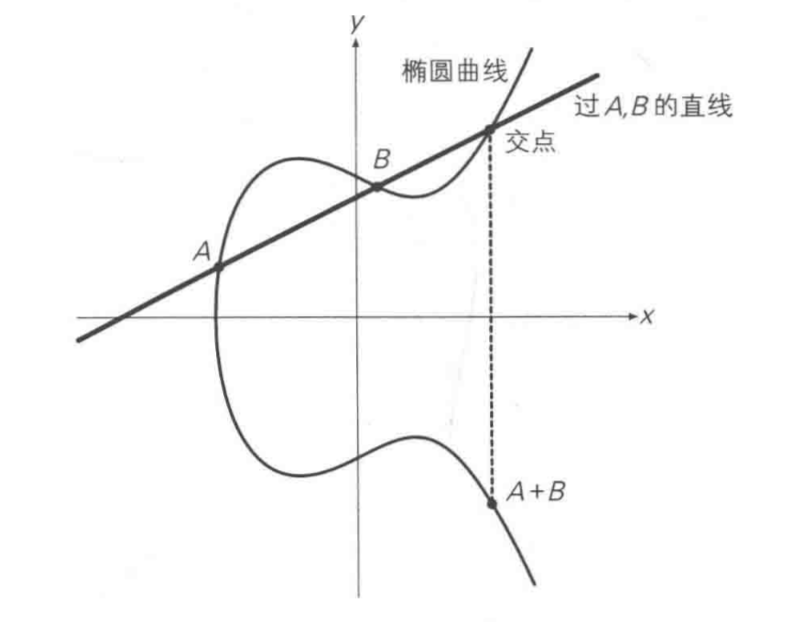过A、B两点的直线，与曲线的交点，关于x轴对称的点为A+B。
对于A+A，即两点重合的情况，则是取A点的切线：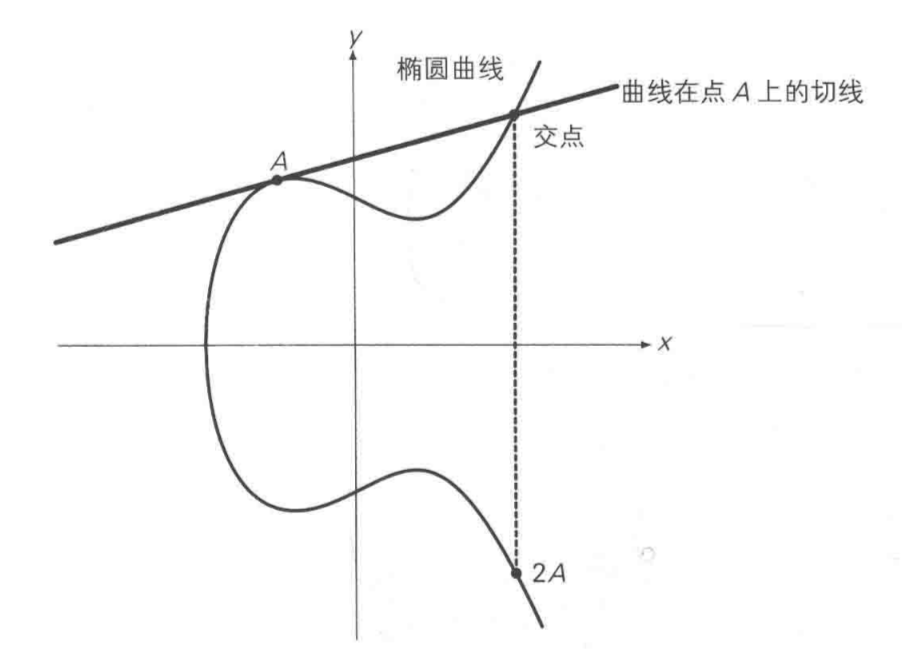将A关于x轴对称位置的点定义为-A，即椭圆曲线的正负取反运算：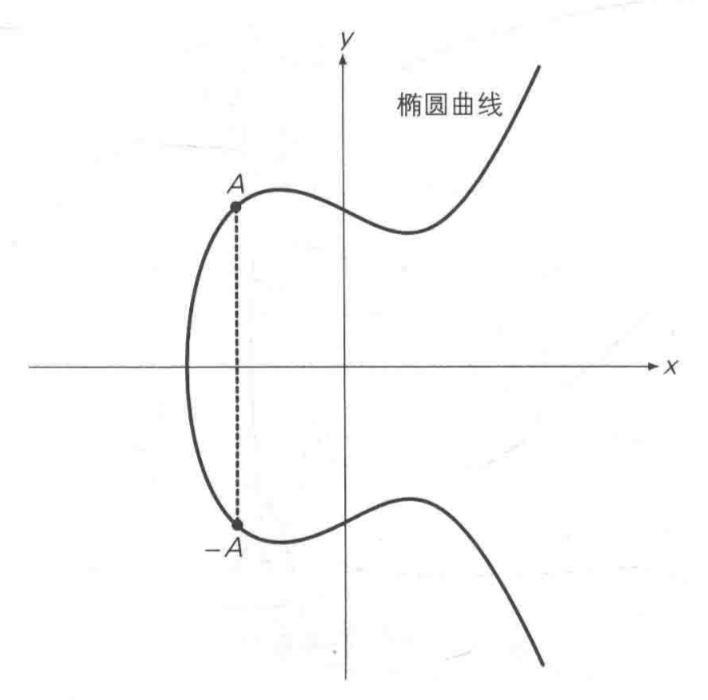综上，给定椭圆曲线上的某一个点G，可以计算出2G=G+G，3G=G+2G，…，以此类推。即给定G点，已知k，就可以计算出kG；而已知kG，却很难计算出k值，这是离散对数困难问题。这就符合非对称加密的特点，私钥可以推出公钥，公钥不能推出私钥，其中，大数k就为私钥，kG即为公钥。
2 椭圆曲线加解密算法
已知：给定G点，私钥k，公钥P=kG
公钥加密：对消息m进行加密，生成随机数 r，密文C={rG，m+rP}，
私钥解密：m+rP - k(rG) = m + rP - r(kG) = m
注：公布的是rG，而不是r，根据rG很难算出r。随机数r不可暴露，否则rP可被计算出，起不到加密效果。
3 椭圆曲线签名算法
椭圆曲线签名算法有很多种，这里以Schnorr签名为例。
3.1 签名过程
签名其实就是为了向验证方保证这是我发送的消息，但又不能暴露私钥。
从最原始的公私钥关系开始推起：P = kG。
比如我要对m消息，做个签名，我可以这样吗：
计算出
$e = hash(m)$
$s = ke$
然后把 消息m 和 签名s 发送给对方，对方收到后，计算：
$S_1 = sG$
$S_2 = eP$
因为s、G、P，都是已知的，e可以根据m计算出，所以S1和S2都可以很快计算出，计算之后，只需要验证S1=S2即可，原理：
$sG = (ke)G = e(kG) = eP$
是不是非常巧妙？

但是其中问题非常明显，眼尖的朋友可能早就可以看出，s = ke，e是可以计算出的，把s发送出去之后，明显私钥k也可以很容易计算出来：
$k = s/e$
这是简单的除法运算。
那怎么解决这个问题呢？其实问题就出在s = ke里面只有一个未知数k，如果再引入一个未知数，k就无法被计算出来了：
$s = r + ke$
其中r是可以是一个随机数。
但这样的话，接收方又要怎么验证呢？
试试：
sG = (r + ke)G = rG + eP
可见，还需要rG的值才可以验证，记R = rG。这同样利用了椭圆曲线的性质，已知rG很难计算出r。
所以，签名就包含两个部分，一个由随机数r计算出的点R，还有由随机数r、私钥k和消息哈希e计算出的s，记为<R, s>，其中：
$r = random$
$e = hash(m)$
$R = rG$
$s = r + ke$

这样，是不是就没问题了呢？
我们来看一下，假如我们对同一个数据m，进行两次签名：
$s_1 - s_2 = r_1 - r_2 + k(e_1 - e_2)$
因为是同一个数据，按照上面的计算，$e_1 = e_2$，此时：
$s_1 - s_2 = r_1 - r_2$
因为s是签名的一部分，是已知的，那么$r_1$和$r_2$的差值就可以被计算出来，从而可能推算出随机数的计算方法，一旦随机数可预测，则引入的这个随机数自然也起不到作用了。
当然，这可以解决，有两种方法：
① $e = hash(R, m)$
e的计算增加元素R，就会使得即使对于同一个消息m，计算出的e也不一样，就不会有上述问题。
②$r = hash(k, m)$
这种方式计算出的伪随机数，对于同一个私钥对同一个消息签名，生成的r是一样的，就不会有什么随机数会被预测的问题，并且由于私钥对外是未知的，所以r也无法计算得出。

问题：随机数可以每次都一样吗？即固定一个随机数，不对外公布即可。
对于这个问题，我们看一下，假如我们对两条不同的消息进行签名：
$s_1 - s_2 = r_1 - r_2 + k(e_1 - e_2)$
假如我们用的是同一个随机数，即$r_1 = r_2$，那么：
$s_1 - s_2 = k(e_1 - e_2)$
此时s是已知的，e也可以计算出来，从而私钥也可以计算得出，所以随机数一定不能每次都一样，但如果为了实现同一个私钥对同一条消息每次签名结果都一样，那么就可以使用上面的方法②$r = hash(k, m)$，这样既可以保证对同一条消息生成一样的r，又能保证消息不同时r也不同。

总结：为了同一私钥对同一消息生成签名相同，我们选用方式②，并总结下签名流程：
①$r = hash(k, m)$
②$e = hash(m)$
③$R = rG$
④$s = r + ke$
⑤签名<$R, s$>
3.2 验签过程
收到消息m和签名<R, s>，已知发送方公钥P，验证如下：
①$e = hash(m)$
②$S_1 = sG$
③$S_2 = R + eP$
④验证$S_1 = S_2$
4 聚合签名
聚合签名是指多个签名方对多条消息或者同一消息进行签名。最简单的方式就是生成多个签名，验证的时候再一个一个验证，但既然提出聚合签名这个概念，就说明有更好的方式进行验证。Schnorr签名由于其线性的特征，很容易改造成聚合签名，线性是因为这个式子：
$s = r + ke$
以及椭圆曲线上的运算律：
$xG + yG = (x+y)G$
上述公式是一种完美的线性特性，椭圆曲线在Mod 有限域的这种特性，是很多的Crypto机制的基础。
我们来看看验证n个签名时的计算：
①$e_1 = hash(m_1)$
②$S_1^1 = s_1G$
③$S_2^1 = R_1 + e_1P_1$
④验证$S_1^1 = S_2^1$
①$e_2 = hash(m_2)$
②$S_1^2 = s_2G$
③$S_2^2 = R_2 + e_2P_2$
④验证$S_1^2 = S_2^2$
…
①$e_n = hash(m_n)$
②$S_1^n = s_nG$
③$S_2^n = R_n + e_nP_n$
④验证$S_1^n = S_2^n$
有没有一种方式，可以减少计算量？答案是有的。试试把所有$S_1$和$S_2$分别相加：
$sum(S_1)^i = s_1G + s_2G + ... + s_nG = (s_1 + s_2 + ... + s_n)G$
$sum(S_2)^i = (R_1 + R_2 + ... + R_n) + (e_1P_1 + e_2P_2 + ... + e_nP_n)$
对于$S1$，进行结合之后，原本 n 次的点乘和 n-1 次点的加法，变成了 n-1 次的大数加法和 1 次点乘。加法的复杂度相对点乘的复杂度可以忽略不计，所以近似为n次点乘减少到1次点乘。
对于S2，看起来并没有计算次数上的减少，但这种结合性，使得多签可以表示成跟单签一样的形式：
<$R_1 + R_2 + ... + R_n, s_1 + s_2 + ... + s_n$>
同样是一个点加一个大数的形式。
此外，当多签的是同一条消息时，e相同：
$sum(S_2)^i = (R_1 + R_2 + ... + R_3) + e(P_1 + P_2 + ... + P_n)$
这样又减少了很多次点乘。
5 密钥消除攻击
看这种情况：

张三公钥为P1 ，对某个消息（不一定是m）的签名为<$R_1, s_1$>
李四公钥为P2，但他对外公布自己公钥为$P_2^1 = P_2 - P_1$，对消息m的签名为<$R_2 - R_1, s_2 - s_1$>，记为<$R_2^1, s_2^1$>
聚合签名则为：<$R_1+R_2^1, s_1+s_2^1$>
有$(s_1+s_2^1)G = (s_1 + s_2 - s_1)G = s_2G = (r_2 + k_2e)G = R_2 + eP_2 = (R_1+R_2^1) + e(P_1 + P_2^1)$

而验证方恰恰只验证$(s_1+s_2^1)G = (R_1+R_2^1) + e(P_1 + P_2^1)$，根据上面的推理，这当然是成立的，问题就在于李四没有诚实公布自己的公钥，并且对签名结果做了处理。这个问题的后果是，李四只需要取到张三对任何一个消息的签名，就可以拿这个签名到处伪造其他消息的聚合签名，而张三完全不知情，这是致命的。
解决这个问题，大致思路就是，设法让签名者无法构造一个假的公钥，或者证明这个公钥确实是他的。已经有了一个现成的方法可以解决，Musig：
可以参考这篇文章的描述：Schnorr 签名介绍
这个思路是所有签名方的公钥做一个运算，形成一个共享的公钥，这样，如果一个人想伪造一个公钥，得先获得这个共享公钥，但这个共享公钥的计算又依赖于他公布的公钥，所以想伪造一个合适的公钥是不可能的。


展开全文• 原文链接如下： Elliptic Curve Cryptography: ECDH and ECDSA​andrea.corbellini.name想全面了解椭圆曲线的朋友可以先看看前两个部分，翻译得很棒：Avery：ECC椭圆曲线加密算法：介绍​zhuanlan.zhihu.comAvery：...
• 对于有兴趣研究椭圆曲线加密算法及硬件实现的朋友们非常有帮助
• 1985 年，Miller 和 Koblitz 各自独立的提出了椭圆曲线公钥密码，它是基于有限域上椭圆曲线构成加密体制，其安全性基于有限域上椭圆曲线离散对数问题（Elliptic Curve Discrete Logarithm Problem, ECDLP）的难解...
• 椭圆曲线加密算法 椭圆曲线：E:y2=ax3+ bx2+cx+d 假设a=1,b=0,c=-2,d=4时，所得到的椭圆曲线为:E:y2=x3-2x+4 如下图所示 椭圆曲线的运算规则 过曲线上的两点A、B画一条直线，找到直线与椭圆曲线的交点，交点关于x...
• 椭圆曲线加密算法，让大家学习一下！可以写成论文！...